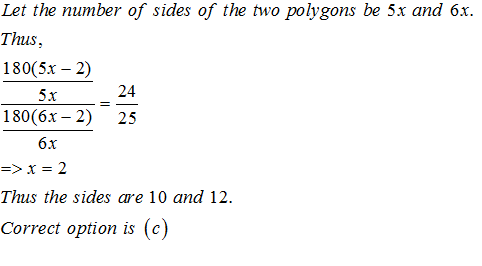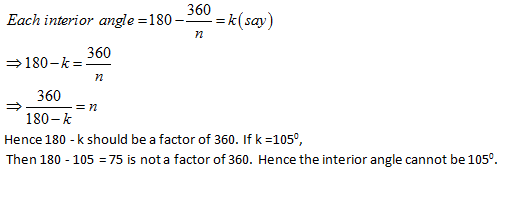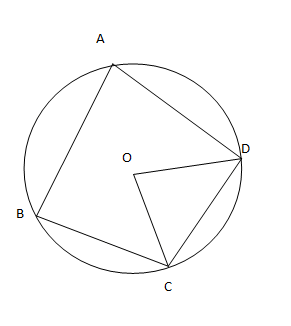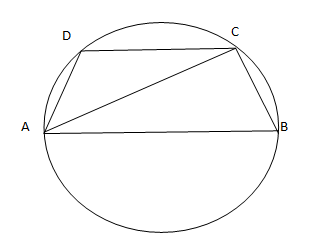• This is an assessment test.
• These tests focus on geometry and mensuration and are meant to indicate your preparation level for the subject.
• Kindly take the tests in this series with a pre-defined schedule.

## Geometry and Mensuration: Test 27

Congratulations - you have completed Geometry and Mensuration: Test 27. You scored %%SCORE%% out of %%TOTAL%%. You correct answer percentage: %%PERCENTAGE%% . Your performance has been rated as %%RATING%%
 Question 1
Each internal angle of regular polygon is two times its external angle. Then the number of sides of the polygon is:
 A 8 B 6 C 5 D 7
Question 1 Explanation:
The interior angle + exterior angle =180o
Thus the interior angle = 120o and each exterior angle  = 60o
The sum of all the exterior angles = 3600
The number of sides = 3600/600 = 6
Correct option is (b)
 Question 2
Ratio of the number of sides of two regular polygons is 5: 6 and the ratio of their each interior angle is 24: 25. Then the number of sides of these tow polygons are
 A 20, 24 B 15, 18 C 10, 12 D 5, 6
Question 2 Explanation:Question 3
Measure of each interior angle of a regular polygon can never be;
 A 150o B 105o C 108o D 144o
Question 3 Explanation:Question 4
ABCD is a cyclic quadrilateral and O is the center of the circle. If ∠COD= 140o and ∠BAC= 40o, then the value of ∠BCD is equal to
 A 70o B 90o C 60o D 80o
Question 4 Explanation:∠COD=140, thus∠ CAD= ½ of ∠COD= 70.
∠BCD= 180-110=70.
Correct option is (a)
 Question 5
ABCD is a cyclic trapezium with AB||DC and AB= diameter of the circle. If ∠CAB= 30o, then ∠ADC is
 A 60o B 120o C 150o D 30o
Question 5 Explanation:Here ∠CAB = 300 and ∠ACB = 900 Hence ∠ABC = 600
Now ∠ADC + ∠ABC = 1800
∠ADC = 1800 - 600 = 1200
Once you are finished, click the button below. Any items you have not completed will be marked incorrect.
There are 5 questions to complete.
 ← List →